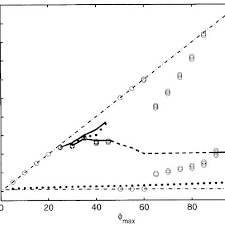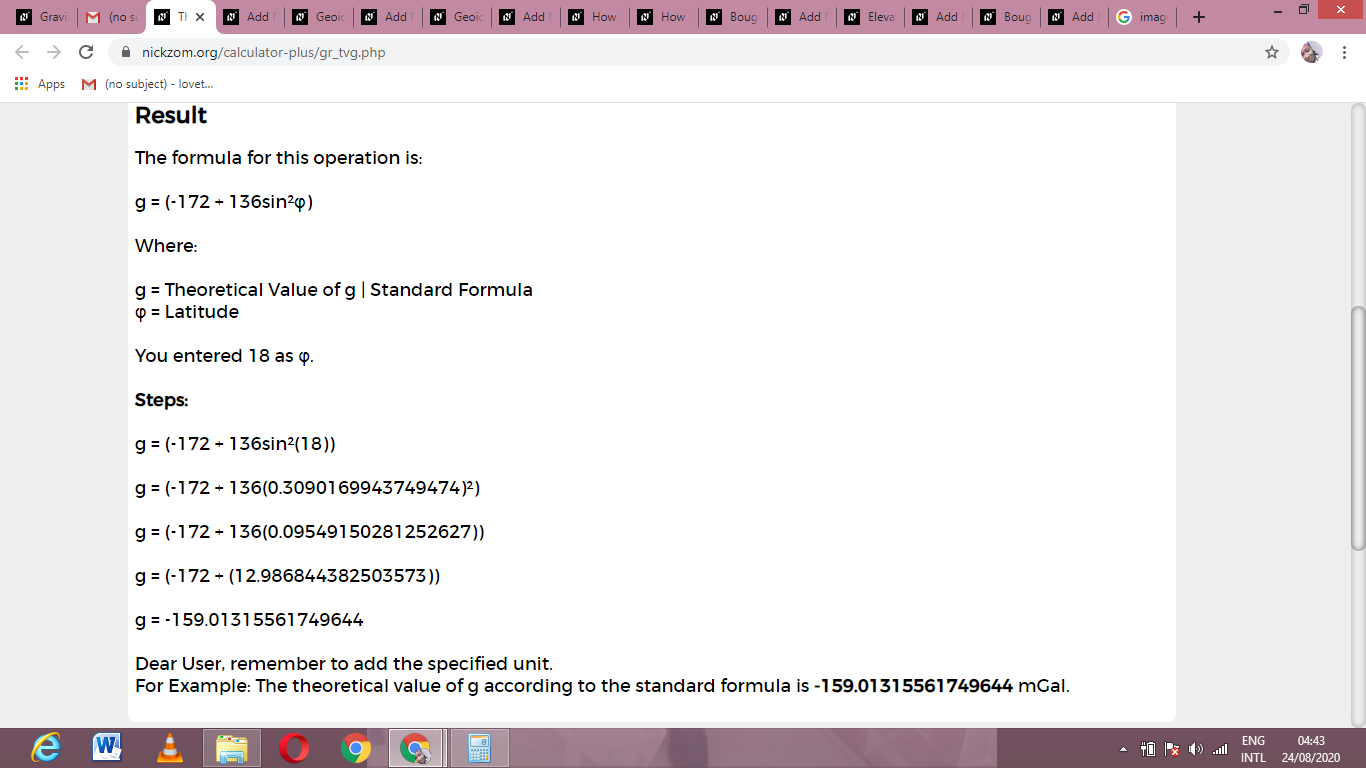# How to Calculate and Solve for Theoretical Value of g | Standard Formula | GravityThe image above represents theoretical value of g.

To compute for theoretical value of g, one essential parameter is needed and this parameter is Latitude (φ).

The formula for calculating theoretical value of g:

g = (-172 + 136sin²φ)

Where:

g = Theoretical Value of g | Standard Formula
φ = Latitude

Let’s solve an example;
Find the theoretical value of g when the latitude is 18.

This implies that;

φ = Latitude = 18

g = (-172 + 136sin²φ)
g = (-172 + 136sin²(18))
g = (-172 + 136(0.309)²)
g = (-172 + 136(0.0954))
g = (-172 + (12.986))
g = -159.01

Therefore, the theoretical value of g is -159.01 mGal.

Nickzom Calculator – The Calculator Encyclopedia is capable of calculating the theoretical value of g.

To get the answer and workings of the theoretical value of g using the Nickzom Calculator – The Calculator Encyclopedia. First, you need to obtain the app.

You can get this app via any of these means:

To get access to the professional version via web, you need to register and subscribe for NGN 2,000 per annum to have utter access to all functionalities.
You can also try the demo version via https://www.nickzom.org/calculator

Apple (Paid) – https://itunes.apple.com/us/app/nickzom-calculator/id1331162702?mt=8
Once, you have obtained the calculator encyclopedia app, proceed to the Calculator Map, then click on Geology under Add-on.Now, Click on Gravity under GeologyNow, Click on Theoretical Value of g under GravityThe screenshot below displays the page or activity to enter your value, to get the answer for the theoretical value of g according to the respective parameter which is the Latitude (φ).Now, enter the value appropriately and accordingly for the parameter as required by the Latitude (φ) is 18.Finally, Click on CalculateAs you can see from the screenshot above, Nickzom Calculator– The Calculator Encyclopedia solves for the theroretical value of g and presents the formula, workings and steps too.